# WEST Math: Area Under the Curve & Integrals Chapter Exam

Exam Instructions:

Choose your answers to the questions and click 'Next' to see the next set of questions. You can skip questions if you would like and come back to them later with the yellow "Go To First Skipped Question" button. When you have completed the practice exam, a green submit button will appear. Click it to see your results. Good luck!

### Page 1

#### Question 1 1. Given f(x) on the graph, what does z equal?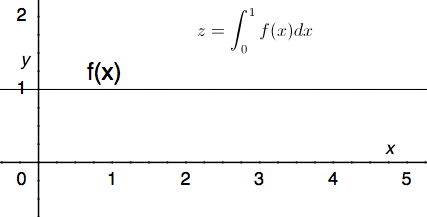#### Question 2 2. The following integral can be re-written as: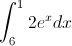#### Question 3 3. Write out the first four terms of a Riemann sum equivalent to the following integral.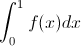#### Question 5 5. Given f(x) on the graph below, which of the following integrals will equal 0?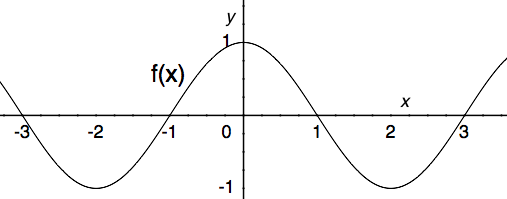### Page 2

#### Question 6 6. Given the following information, what does z equal?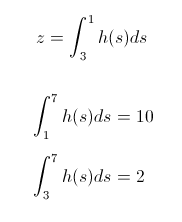#### Question 7 7. In the following equation, s is the _____.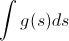#### Question 8 8. Which integral would be evaluated using the following expression (given that F'(x) = f(x))?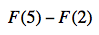#### Question 9 9. Given a continuous function f(x) that satisfies the equation below, which of the following is true, according to the average value theorem?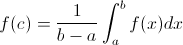#### Question 10 10. Use the average value theorem to determine the average value of f(x) between 0 and 2.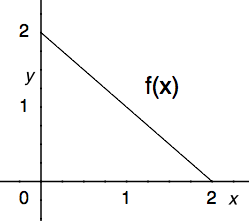### Page 3

#### Question 11 11. Which of the following expressions is equal to the integral below?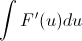#### Question 12 12. Which of the following integrals represents the area under the graph below?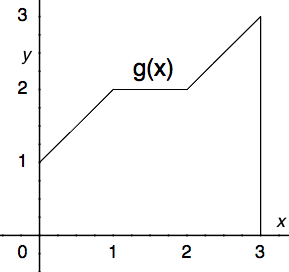#### Question 13 13. Evaluate the following integral.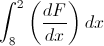#### Question 14 14. Which of the following integrals is equal to the following summation over the continuous region x=a to x=b?#### Question 15 15. Use the fundamental theorem of calculus to solve the problem below.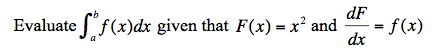### Page 4

#### Question 16 16. The average value for this continuous function over the shaded region is 2. According to the average value theorem, how many times does this function pass through the value 2?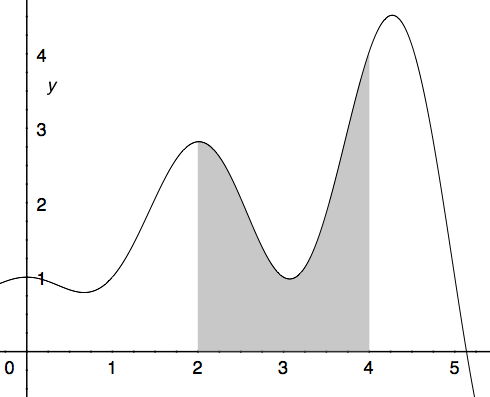#### Question 17 17. What is the one term left-sided Riemann Sum approximation for the integral below?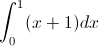#### Question 18 18. Which of the following shows the correct method for evaluating the integral below?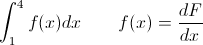#### Question 19 19. In the following equation, g(s) is the _____.#### Question 20 20. How many terms are in the Riemann sum represented below?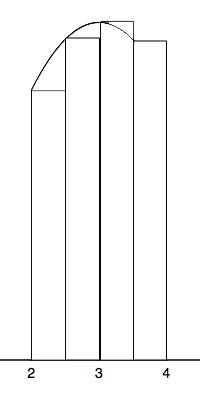### Page 5

#### Question 21 21. Using the single term Riemann sum as shown, what is the area beneath the curve f(x) between 0 and two pi?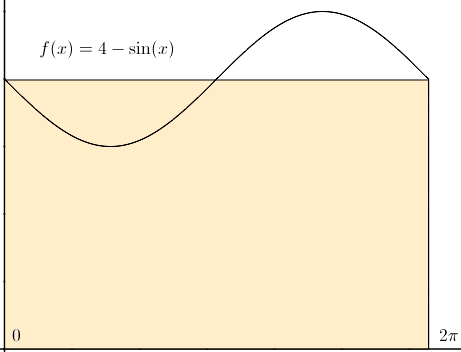#### Question 22 22. Which of the following can NOT be a solution for the integral below?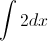#### Question 23 23. Use the fundamental theorem of calculus to evaluate the following integral.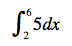#### Question 24 24. Estimate the area beneath the curve f(x) using the two term Riemann sum as shown below.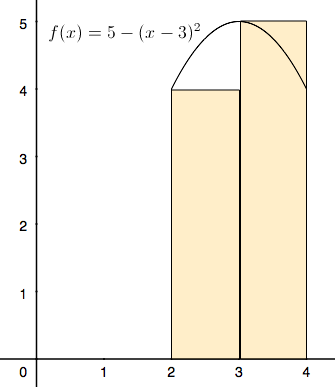#### Question 25 25. Which of the following sums represents an n-term Riemann sum for the integral below?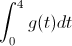### Page 6

#### Question 26 26. You are going to take a Riemann Sum of the area below. To do this, you divide the region into four slices of equal width. How wide is each slice?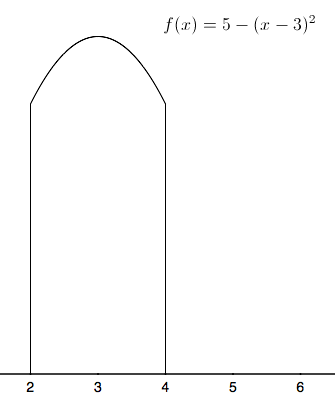#### Question 27 27. Evaluate the following.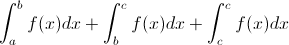#### Question 29 29. Which integral below is equal to the following integral?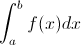#### Question 30 30. Which of the following is a representation of a Riemann sum estimation of the following integral?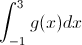#### WEST Math: Area Under the Curve & Integrals Chapter Exam Instructions

Choose your answers to the questions and click 'Next' to see the next set of questions. You can skip questions if you would like and come back to them later with the yellow "Go To First Skipped Question" button. When you have completed the practice exam, a green submit button will appear. Click it to see your results. Good luck!

Support